Courses

# General Intelligence & Reasoning - 1 MCQ

## 50 Questions MCQ Test Mock Test Series for SSC JE Mechanical Engineering | General Intelligence & Reasoning - 1 MCQ

Description
This mock test of General Intelligence & Reasoning - 1 MCQ for SSC helps you for every SSC entrance exam. This contains 50 Multiple Choice Questions for SSC General Intelligence & Reasoning - 1 MCQ (mcq) to study with solutions a complete question bank. The solved questions answers in this General Intelligence & Reasoning - 1 MCQ quiz give you a good mix of easy questions and tough questions. SSC students definitely take this General Intelligence & Reasoning - 1 MCQ exercise for a better result in the exam. You can find other General Intelligence & Reasoning - 1 MCQ extra questions, long questions & short questions for SSC on EduRev as well by searching above.
QUESTION: 1

### In the following question, select the related word from the given alternatives. Scissors : Cloth :: Axe : ?

Solution:

As scissors is used for cutting the clothes,

Similarly,

Axe is used for cutting wood.

Hence, the correct answer is wood.

QUESTION: 2

### In the following question, select the related word from the given alternatives. Fan : Blades : : ? : ?

Solution:

Fan contains blades as its major component. Similarly, Book contains chapters as its major component.

Hence, Book : Chapter is the correct ans.

QUESTION: 3

### In question, select the related word from the given alternatives. Elated : Despondent :: Enlightened : ____

Solution:

Elated means happy which is the opposite of despondent that means discourage. Similarly, enlightened is the opposite of ignorant.

QUESTION: 4

In question below, select the related letters from the given alternative.

BYCX :DWEV :: FUGT : ?

Solution:
QUESTION: 5

Select the related letters from the given alternatives.

DBCE: QOPR :: JLKI :?

Solution:
QUESTION: 6

Directions: In the following question, select the odd letters from the given alternatives.

ACEG : IKMO :: QSUW : ?

Solution:
QUESTION: 7

In question select the related numbers from the given alternatives.

76 : 01 :: 42 : ?

Solution:

The difference between the first two digits gives the second number.

For example, 7 – 6 = 1.

Similarly, 4 – 2 = 2.

Therefore, the final pairing will be 76 : 01 ∷ 42 : 2.

QUESTION: 8

Select the related numbers from the given alternatives :
35 : 48 :: 110 : ?

Solution:

In 35 : 48

7 × 5 = 35

8 × 6 = 48

Similarly;

11 × 10 = 110

12 × 11 = 132

Hence, 132 is correct option.

QUESTION: 9

Select the related number from the given alternatives.

36 : 25 : : 100 : ?

Solution:

In 36 : 25

⇒ 62 : 52

⇒ 62 : (6 – 1)2

Similarly,

⇒ 100 : ?

⇒ 102 : (10 – 1)2

⇒ 102 : 92

100 : 81

Hence, “81” is required number.

QUESTION: 10

Find the odd word from the given alternatives.

Solution:

Square, Rectangle and Circle are 2-dimensional figures while Sphere is a 3-dimensional figure.

QUESTION: 11

Directions: Select the one which is different from the other three responses.

Solution:

Ear, Eye and Nose are the sensory organs but neck is not.

Hence Neck is different from other responses.

QUESTION: 12

In the following question, select the related word pair from the given alternatives.

Rabbit : Animal : : ? : ?

Solution:

Just as Rabbit is an animals, Pentagon is a Figure.

Hence, answer is Pentagon: Figure.

QUESTION: 13

Select the odd letters from the given alternatives.

Solution:

OP, IJ, DE are consecutive letter in sequence but VU are consecutive letter but not in sequence.

Therefore, VU is odd one out.

QUESTION: 14

Direction: Select the one which is different from the other three responses :

Solution:

ABCD is continuous series while in other series there are having some missing letters in between.

QUESTION: 15

Directions: Find the odd letters from the given alternatives.

Solution:

M + 1 = N, D + 1 = E

H + 1 = I, X + 1 = Y

L + 1 = M, V + 1 = W

R – 1 = Q, C – 1 = B

The odd one out is RQCB.

QUESTION: 16

Direction: In the question given find the odd number from the given alternatives.

Solution:

Here, 976, 778 and 895 are consecutive number whereas 997 is a prime number.

Therefore, “997” is odd one among given alternatives.

QUESTION: 17

Find the odd number pair from the given alternatives.

Solution:

The digits of the given pairs of numbers are in reverse order of each other.

Hence odd should be 96-19 because instead of 19 it should be 69.

QUESTION: 18

Find the odd number pair from the given alternatives.

Solution:

Here the pattern is : Number - Number × 2.

36 ­ 72 → 36 - 36 × 2 = 72

17 ­ 34 → 17 - 17 × 2 = 34

28 ­ 49 → 28 - 28 × 2 = 56

24 ­ 48 → 24 - 24 × 2 = 48

Thus 28 - 49, is the odd one out.

QUESTION: 19

Which of the following terms follows the trend of the given list?

YXXXXXX, YYXXXXX, YYYXXXX, YYYYXXX, YYYYYXX, _______________.

Solution:

The pattern here is the letter ‘X’ which is to the immediate right of letter ‘Y’ is converted to ‘Y’ in every step and hence increasing the number of letter ‘Y’ in every step i.e.

YXXXXXX, YYXXXXX, YYYXXXX, YYYYXXX, YYYYYXX, YYYYYYX.

Hence, YYYYYYX is the correct alternative.

QUESTION: 20

A series is given with one term missing. Select the correct alternative from the given ones that will complete the series.

YZ, VW, ST, ?, MN

Solution:
QUESTION: 21

A series is given with one term missing. Select the correct alternative from the given ones that will complete the series.

POT, OMS, NKR, MIQ, ?

Solution:

P – 1 = O, O – 1 = N, N- 1 – M, M- 1 = L

O – 2 = M, M- 2 = K, K – 2 = I, I – 2 = G

T – 1 = S, S- 1 = R, R- 1 = Q, Q – 1= P

Hence LGP is the correct answer.

QUESTION: 22

A series is given with one term missing. Select the correct alternative from the given ones that will complete the series.

RMT, PKR, NIP, ?, JEL

Solution:
QUESTION: 23

In the following question, select the missing number from the given series.

18, 14, 10, 6, ?

Solution:

The relation between the numbers is as follows,

18 - 4 = 14

14 - 4 = 10

10 - 4 = 6

Hence, the next number is 6 - 4 = 2.

QUESTION: 24

A series is given, with one number missing. Choose the correct alternative from the given ones that will complete the series.

-4, ?, 1, 3.5, 6, 8.5

Solution:

The pattern followed is: (+ 2.5) is added in each number.

→ -4 + 2.5 = -1.5

→ -1.5 + 2.5 = 1

→ 1 + 2.5 = 3.5

→ 3.5 + 2.5 = 6

→ 6 + 2.5 = 8.5

→ 8.5

Hence, the missing number is 8.5 in the given series.

QUESTION: 25

Replace the “?” in the following series.

7, 7, 14, 42, ?, 840

Solution:

7

7 x 1= 7

7 x 2 x 1= 14

7 x 3 x 2 x 1 = 42

7 x 4 x 3 x 2 x 1 = 168

7 x 5 x 4 x 3 x 2 x 1 = 840

Hence missing number is 168.

QUESTION: 26

In a certain code language, 462 means 'lion is wild', 543 means 'cat is hungry', 165 means 'wild and hungry'. Find the code for 'and'.

Solution:
QUESTION: 27

If ANTIQUES is coded as DQWLTXHV, then how will FOX be coded as?

Solution:

ANTIQUES is coded as DQWLTXHV

⇒ A + 3 = D

⇒ N + 3 = Q

⇒ T + 3 = W

⇒ I + 3 = L

⇒ Q + 3 = T

⇒ U + 3 = X

⇒ E + 3 = H

⇒ S + 3 = V

Similarly,

⇒ F + 3 = I

⇒ O + 3 = R

⇒ X + 3 = A

Hence, in this language “FOX” will be coded as “ IRA”.

QUESTION: 28

Ram walked 20 m towards the north. Then he turned right and walks 30 m. Then he turns right and walks 35 m. Then he turns left and walks 15 m. Finally he turns left and walks 15 m. In which direction and how many metres is he from the starting position?

Solution:
QUESTION: 29

Pointing to a lady in the photograph, Sakshi said, ‘Her son’s father is the son-in-law of my mother”. How is the lady related to sakshi?

Solution:
QUESTION: 30

Shyama, a woman, says that father of Rajiv’s father is my father. How Shyama is related to Rajiv?

Solution:

Shyama, a woman, says that father of Rajiv’s father is my father.

Now, father of Rajiv’s father is Rajiv’s grandfather.

That means Shyama’s father is Rajiv’s grandfather.

Thus Syama is Rajiv’s aunt.

QUESTION: 31

If ‘A + B’ stands for ‘A is the father of B’, ‘A – B’ stands for ‘A is the brother of B’, ‘A × B’ stands for ‘A is the wife of B’ and ‘A ÷ B’ stands for ‘A is the sister of B’ then ‘P + Q ÷ R’ means ?

Solution:

Given conditions is: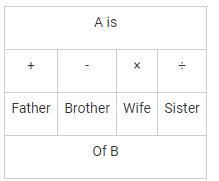Now, P + Q ÷ R means

P is father of Q and Q is sister of R.

This means P is father of R is true & other options are irrelevant as Gender of R can’t be determined with the given data.

QUESTION: 32

Arrange the given words in the sequence in which they occur in the dictionary.

1. Counter

2. Crop

3. Create

4. Carnation

5. Creator

Solution:

4. Carnation

1. Counter

3. Create

5. Creator

2. Crop

Hence, correct dictionary order is 41352.

QUESTION: 33

Arrange the following in dictionary order. Then which will be the second word?

1) Specific

2) Spectacle

3) Specified

4) Spectacular

Solution:

In dictionary order, words are put in alphabetically according to the first letter in which they differ.

Thus, the order will be:

Specifi(1), Specified (3), Spectacle (2), Spectacular (4)

QUESTION: 34

Directions: In the following questions, which one of the following words cannot be formed form the letters of the given word (using each letter once)?

VACCINATION

Solution:

1. VACATION = can be formed as VACCINATION

2. NATION = can be formed as VACCINATION

3. CIVIC = can be formed as VACCINATION

4. NICE = cannot be formed as E is not present in VACCINATION

QUESTION: 35

In the following question below are given some statements followed by some conclusions. Taking the given statements to be true even if they seem to be at variance from commonly known facts, read all the conclusions and then decide which of the given conclusion logically follows the given statements.

Statements:

I. Some pen are black.

II. Some pen are blue.

III. No black is red.

Conclusions:

I. No pen is red.

II. Some blue are black.

III. Some black are pens.

Solution:
QUESTION: 36

Given below are two statements followed by two conclusions. Assuming these statements to be true, decide which one logically follow.

Statements:

I. All film stars are playback singers

II. All film directors are film stars.

Conclusions:

I. All film directors are playback singers

II. Some film star are film directors

Solution:
QUESTION: 37

Select the missing number in the pattern from the given responses.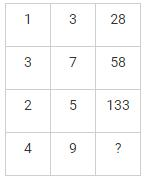Solution:

1st row = 13 + 3= 1 + 27 = 28

2nd Row = 32 + 72 = 9 + 49 = 58

3rd row = 23 + 53 = 8 + 125 = 133

4th row = 42 + 92 = 16 + 81 = 97

Therefore 97 is odd one among them.

QUESTION: 38

Some friends went out for a walk. Divya walked before farhan. Sumit was walking behind mona and ahead of farhan. Who walked last?

Solution:

According to the question, Divya walked before farhan

Divya ← Farhan

Also, Sumit was walking behind mona and ahead of farhan

Mona ← Sumit ← Farhaan

Combining both the situation we get;

Mona ← Sumit ← Divya ← Farhaan

Hence, Farhaan is walking at last

QUESTION: 39

If ‘ + ’ means ‘multiplication, ‘ - ’ means ‘division’, ‘×’ means ‘subtraction’ and ‘÷’ means ‘addition’, then 9 + 8 ÷ 8 – 4 × 9 is

Solution: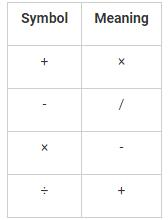We use BODMAS to calculate the following expression.

9 + 8 ÷ 8 – 4 × 9 = 9 × 8 + 8/4 – 9 = 9 × 8 + 2 – 9 = 72 + 2 – 9 = 74 – 9 = 65.

Therefore, 65 is the correct answer.

QUESTION: 40

If 18 × 12 = 206 and 19 × 22 = 408, then

23 × 36 = ?

Solution:

The pattern followed here is:

In 18 × 12 = 206 → 18 × 12 = 216, 216 - 10 = 206

In 19 × 22 = 408 → 19 × 22 = 418, 418 - 10 = 408

Hence, In 23 x 36 = ?

23 × 36 = 828, 828 - 10 = 818

Hence, answer is 818.

QUESTION: 41

Which one of the following figures represents the relationship among Shirts, Bed sheets and Towels?

Solution:
QUESTION: 42

Study the following figure and answer the question given below.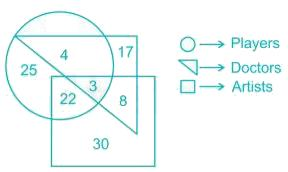How many artists are players?

Solution:

Square represents artists.

Triangle represents doctors.

Circle represents players.

Therefore, the number of artists who are players = 22 + 3 = 25.

QUESTION: 43

Directions: In question, which among the answer figures can be constructed from the parts given in question figure?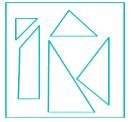Solution:

Option (C) and (D) are incorrect because they do not contain all the parts of the question figure. Option (A) triangles are not in proportion as in question figure. Hence answer is (B).

QUESTION: 44

If a mirror is placed on the line AB, then which of the answer figures is the water image of the given figure?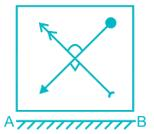Solution:
QUESTION: 45

From the given figures, select the one in which the question figure is hidden/embedded.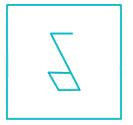Solution:
QUESTION: 46

Identify the figure that completes the pattern.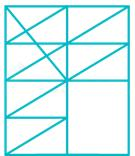Solution:
QUESTION: 47

Which answer figure will complete the pattern in the question figure?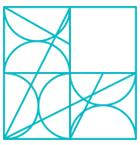Solution:
QUESTION: 48

Which of the following cube in the answer figure cannot be made based on the unfolded cube in the question figure?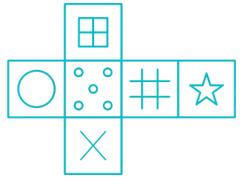Solution:
QUESTION: 49

How many quadrilaterals can be found out from the following figure?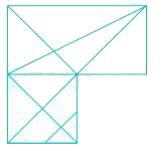Solution:
QUESTION: 50

In the question, a word is represented by only one set of numbers as given in any one of the alternatives. The sets of numbers given in the alternatives are represented by two classes of alphabets as in two matrices given below. The columns and rows of Matrix I are numbered from 0 to 4 and that of Matrix II are numbered from 5 to 9. A letter from these matrices can be represented first by its row and next by its column, e.g., 'R' can be represented by 55, 67 , etc., and 'E' can be represented by 11, 22, etc.

Similarly, you have to identify the set for the word 'COUNT'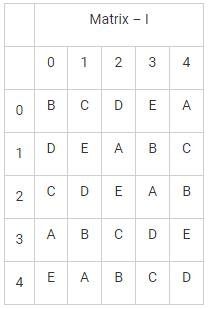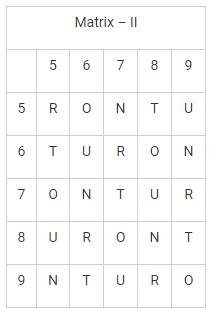Solution:

Let’s check each option

1. 20, 76, 88, 59, 75 represents CNNUO. Hence, definitely not true.

2. 20, 68, 66, 76, 79 represents COUNR. Hence, definitely not true.

3. 32, 56, 66, 88, 89 represents COUNT. Hence, true.

4. 75, 12, 89, 98, 42 represents OATRB. Hence, definitely not true.

So 32, 56, 66, 88, 89 is the correct answer.